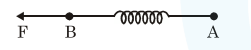# Solve this followingQuestion:

Two masses $A$ and $B$, each of mass $M$ are fixed together by a massless spring. A force acts on the mass $\mathrm{B}$ as shown in figure. If the mass A starts moving away from mass B with acceleration 'a', then the acceleration of mass B wil be :-1. $\frac{\mathrm{Ma}-\mathrm{F}}{\mathrm{M}}$

2. $\frac{\mathrm{MF}}{\mathrm{F}+\mathrm{Ma}}$

3. $\frac{\mathrm{F}+\mathrm{Ma}}{\mathrm{M}}$

4. $\frac{\mathrm{F}-\mathrm{Ma}}{\mathrm{M}}$

Correct Option: , 4

Solution:

$a_{c m}=\frac{m_{1} a_{1}+m_{2} a_{2}}{m_{1}+m_{2}}$

$\frac{F}{2 M}=\frac{M a+M a_{B}}{2 M}$

$a_{B}=\frac{F-M a}{M}$